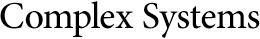## A General Discrete Velocity Model Including Internal Degrees of Freedom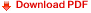H. Nievoll
P. Griehsnig
P. Reiterer
F. Schürrer
Institute for Theoretical Physics,
Technical University of Graz,
Petersgasse 16, A-8010 Graz, Austria

#### Abstract

This paper deals with the problem of describing spatially inhomogeneous gases by applying model Boltzmann equations. A plane discrete velocity model is presented, capable of describing a gas of particles with internal degrees of freedom. The number of particle speeds is variable. All possible collisions, both elastic and inelastic, are calculated. The corresponding equation system for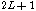speeds is given explicitly, where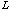is a nonnegative integer. For the case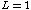solutions in the form of shock waves are found numerically.